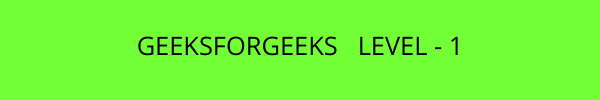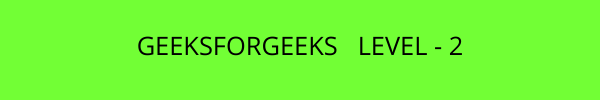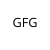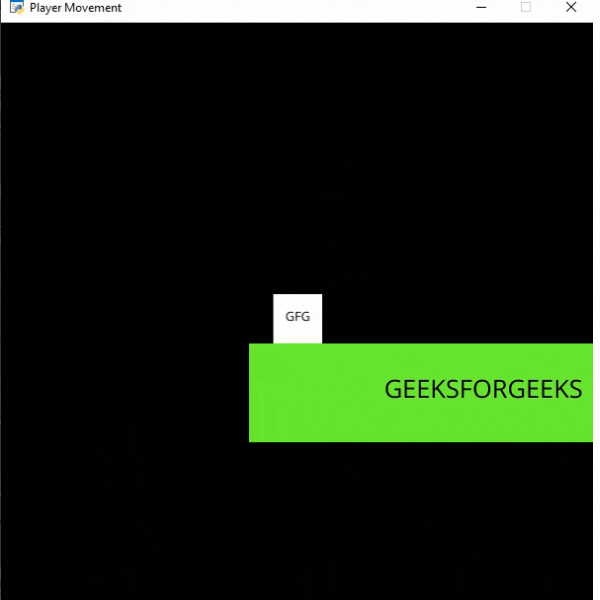Open in App
Not now

• Difficulty Level : Hard
• Last Updated : 23 Sep, 2021

We can easily add multiple levels to our Arcade game by following the below steps:

• Create a new variable to store the current level.
`self.level = 1`
• Load the sprites you are going to use in the current level with the help of self.level variable. For this example, we are going to load ‘Platform1’ named sprites for level 1, ‘Platform2’ named sprite for level 2, and so on.  For this, we can load the platforms using the below code.
`platform = arcade.Sprite(f"Platform{self.level}.png", 1)`
• If the player crosses the right side of the screen then we are going to increase the value of self.level variable to go to the next level.
``` if self.player_sprite.center_x>690:
self.level += 1```

## Functions Used:

• Camera(): The Camera class is used for controlling the visible viewport.

Syntax: arcade.Camera( width , height, window)

Parameters:

• width: width of the viewport
• height: height of the viewport
• window: Window to associate with this camera
• Scene(): A class that represents a scene object.

Parameters:

• sprite_lists: A list of SpriteList objects
• name_mapping: A dictionary of SpriteList objects
• PhysicsEnginePlatformer(): Simplistic physics engine for use in a platformer.

Parameters:

• player_sprite: sprite of the player
• platforms: The sprites it can’t move through
• gravity: Downward acceleration per frame

### Sprites Used:In the below example, we are going to create a MainGame() class. Inside this class first, we are going to initialize some variables for velocity, camera, level, and player’s sprite then we will create 6 functions inside this class.

• on_draw(): Inside this function, we will use our camera and draw the scene.
• setup(): In this function, we will initialize our camera and scene object then we will load our player and platform’s sprites. After that, we will call the  PhysicsEnginePlatformer() function.
• on_update(): In this function, we will update the x coordinates of the player’s sprite, camera, and physics engine. We will also change our level in this function.
• on_key_press() and on_key_release(): In this function, we will change the value of the velocity variable according to the keyboard key that is pressed or released.
• camera_move(): In this function, we will move our camera according to our player’s current position.

Below is the implementation:

## Python3

 `# Importing arcade module``import` `arcade`` ` `# Creating MainGame class``class` `MainGame(arcade.Window):``    ``def` `__init__(``self``):``        ``super``().__init__(``600``, ``600``,``                         ``title ``=` `"Player Movement"``)`` ` `        ``# Initializing a variable to store``        ``# the velocity of the player``        ``self``.vel_x ``=` `0`` ` `        ``# Creating variable for Camera``        ``self``.camera ``=` `None`` ` `        ``# Creating variable to store current level``        ``self``.level ``=` `1`` ` `        ``# Creating scene object``        ``self``.scene ``=` `None`` ` `        ``# Creating variable to store player sprite``        ``self``.player ``=` `None`` ` `        ``# Creating variable for our game engine``        ``self``.physics_engine ``=` `None`` ` `    ``# Creating on_draw() function to draw on the screen``    ``def` `on_draw(``self``):``        ``arcade.start_render()`` ` `        ``# Using the camera``        ``self``.camera.use()``         ` `        ``# Drawing our scene``        ``self``.scene.draw()`` ` `    ``def` `setup(``self``):``         ``# Initialize Scene object``        ``self``.scene ``=` `arcade.Scene()``         ` `        ``# Using Camera() function``        ``self``.camera ``=` `arcade.Camera(``600``, ``600``)`` ` `        ``# Creating different sprite lists``        ``self``.scene.add_sprite_list(``"Player"``)``        ``self``.scene.add_sprite_list(``"Platforms"``,``                                   ``use_spatial_hash``=``True``)`` ` `        ``# Adding player sprite``        ``self``.player_sprite ``=` `arcade.Sprite(``"Player.png"``, ``1``)`` ` `        ``# Adding coordinates for the center of the sprite``        ``self``.player_sprite.center_x ``=` `64``        ``self``.player_sprite.center_y ``=` `600`` ` `        ``# Adding Sprite in our scene``        ``self``.scene.add_sprite(``"Player"``, ``self``.player_sprite)`` ` `        ``# Adding platform sprite according to level``        ``platform ``=` `arcade.Sprite(f``"Platform{self.level}.png"``, ``1``)``         ` `        ``# Adding coordinates for the center of the platform``        ``platform.center_x ``=` `300``        ``platform.center_y ``=` `32``        ``self``.scene.add_sprite(``"Platforms"``, platform)`` ` `        ``# Creating Physics engine``        ``self``.physics_engine ``=` `arcade.PhysicsEnginePlatformer(``            ``self``.player_sprite, ``self``.scene.get_sprite_list(``"Platforms"``), ``0.5``        ``)`` ` `    ``# Creating on_update function to``    ``# update the x coordinate``    ``def` `on_update(``self``, delta_time):`` ` `        ``# Changing x coordinate of player``        ``self``.player_sprite.center_x ``+``=` `self``.vel_x ``*` `delta_time``         ` `        ``# Updating the physics engine to move the player``        ``self``.physics_engine.update()`` ` `        ``# Calling the camera_move function``        ``self``.camera_move()`` ` `        ``# Changing level if player crosses the right``        ``# end of screen``        ``if` `self``.player_sprite.center_x > ``690``:``            ``self``.level ``+``=` `1``            ``self``.setup()`` ` `    ``# Creating function to change the velocity``    ``# when button is pressed``    ``def` `on_key_press(``self``, symbol, modifier):`` ` `        ``# Checking the button pressed``        ``# and changing the value of velocity``        ``if` `symbol ``=``=` `arcade.key.LEFT:``            ``self``.vel_x ``=` `-``300``        ``elif` `symbol ``=``=` `arcade.key.RIGHT:``            ``self``.vel_x ``=` `300`` ` `    ``# Creating function to change the velocity``    ``# when button is released``    ``def` `on_key_release(``self``, symbol, modifier):`` ` `        ``# Checking the button released``        ``# and changing the value of velocity``        ``if` `symbol ``=``=` `arcade.key.LEFT:``            ``self``.vel_x ``=` `0``        ``elif` `symbol ``=``=` `arcade.key.RIGHT:``            ``self``.vel_x ``=` `0`` ` `    ``def` `camera_move(``self``):``       ` `        ``# Getting the x coordinate for the center of camera``        ``screen_x ``=` `self``.player_sprite.center_x ``-` `\``            ``(``self``.camera.viewport_width ``/` `2``)``         ` `        ``# Getting the y coordinate for the center of camera``        ``screen_y ``=` `self``.player_sprite.center_y ``-` `\``            ``(``self``.camera.viewport_height ``/` `2``)`` ` `        ``# Moving the camera``        ``self``.camera.move_to([screen_x, screen_y])`` ` ` ` `# Calling MainGame class``game ``=` `MainGame()``game.setup()``arcade.run()`

Output:My Personal Notes arrow_drop_up